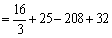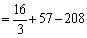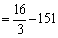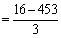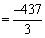# Symbols and notation practice test

1.

(Directions Qn 1 – Qn 4): Read the following  information carefully and answer the questions that follow :

A + B means A is the son of B; A – B means A is the wife of B; A*B means  A is the brother of B; A/B means A is the mother of B and A = B means A is the sister of B.

What does P + R –Q mean ?

Explanation:

Clearly , P + R – Q means P is the son of R who is the wife of Q i.e. Q is the father of P.

2.

What does P*R/Q mean ?

Explanation:

P*R/Q means P is the brother of R who is the mother of Q i.e. P is the uncle of Q.

3.

What does P = R+Q mean ?

Explanation:

P=R+Q means P is the sister of R who is the son of Q i.e. P is the daughter of Q.

4.

What does P = R/Q mean ?

Explanation:

P = R/Q means P is the sister of R Who is the mother of Q i.e. P is the aunt of Q

5.

A \$ B means A is the father of B; A # B means A is the sister of B; A * B means A is the daughter of B and A @ B means A is the brother of B. Which of the following indicates that M is the wife of Q?

Explanation:

Q \$ R → Q is the father of R

R @ T → R is the brother of T Hence, → Q is the father of T T * M → T is the daughter of M Hence, → M is the mother of T Hence, M is the wife of Q.

6.

Choose the correct alternative from the given choices.

If 5@6= 131, 8@9= 299, then 20@21=?

Explanation:

The pattern is 5@6= 5+6=11, 11^2+10=131, …. 20@21= 20+21=41, 41^2+10=1691

7.

If 81 # 145 = 19 and 345 #18 = 21, then what is the value of 98 # 71?

Explanation:

81#145 = (8+1) + (1+4+5) = 9 +10 = 19 Similarly 345 # 18 = (3 + 4 + 5) + (1+8) = 21 :. 98 # 71 = (9+8) + (7+1) = 25

8.

If P stands for ‘addition’ Q stands for ‘subtraction’ R stands for ‘multiplication’ and S stands for ‘division, which of the following statements is correct?

Explanation:

Using the proper notations in (d), we get the statement as 8*8+8/8-8 = 64+1-8 = 57

9.

Which is the third number to the left of the number which is exactly in the middle of the following sequence of numbers ?

1 2 3 4 5 6 7 8 9 2 4 6 8 9 7 5 3 1 9 8 7 6 5 4 3 2 1

Explanation:

Total 27 numbers. So middle number is 14th number = 9.

Third number to the left of this 9 is 4

10.

If ‘a’ means ‘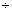‘, ‘b’ means ‘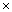‘, ‘c’ means ‘+’, ‘d’ means ‘-‘, then find the value of the expression 72a9b12a18c25d13b16c32 ?

72a9b12a18c25d13b16c32 becomes 7291218+25-1316+32